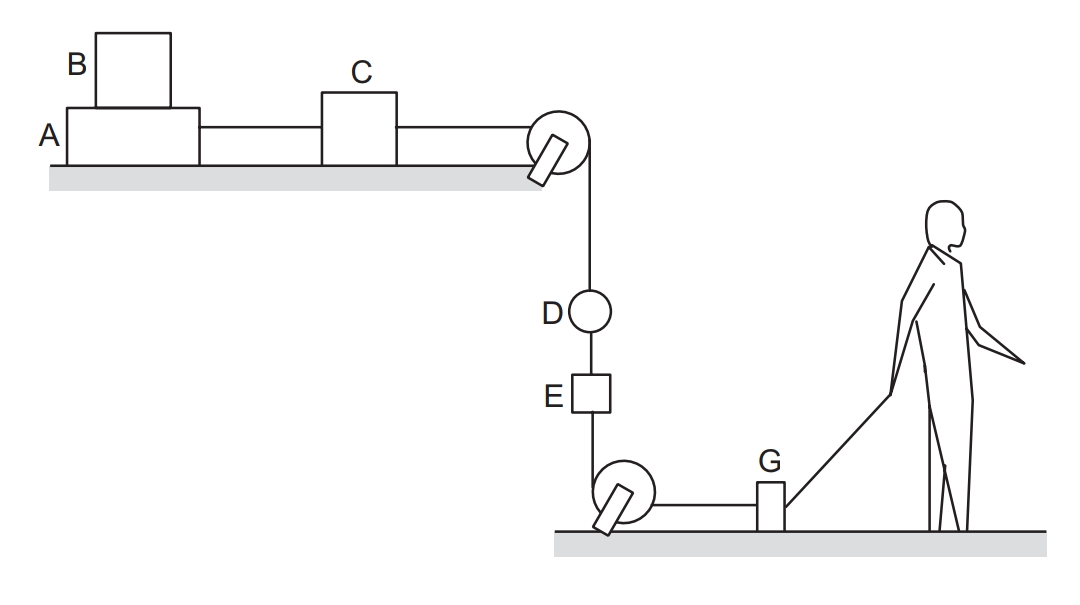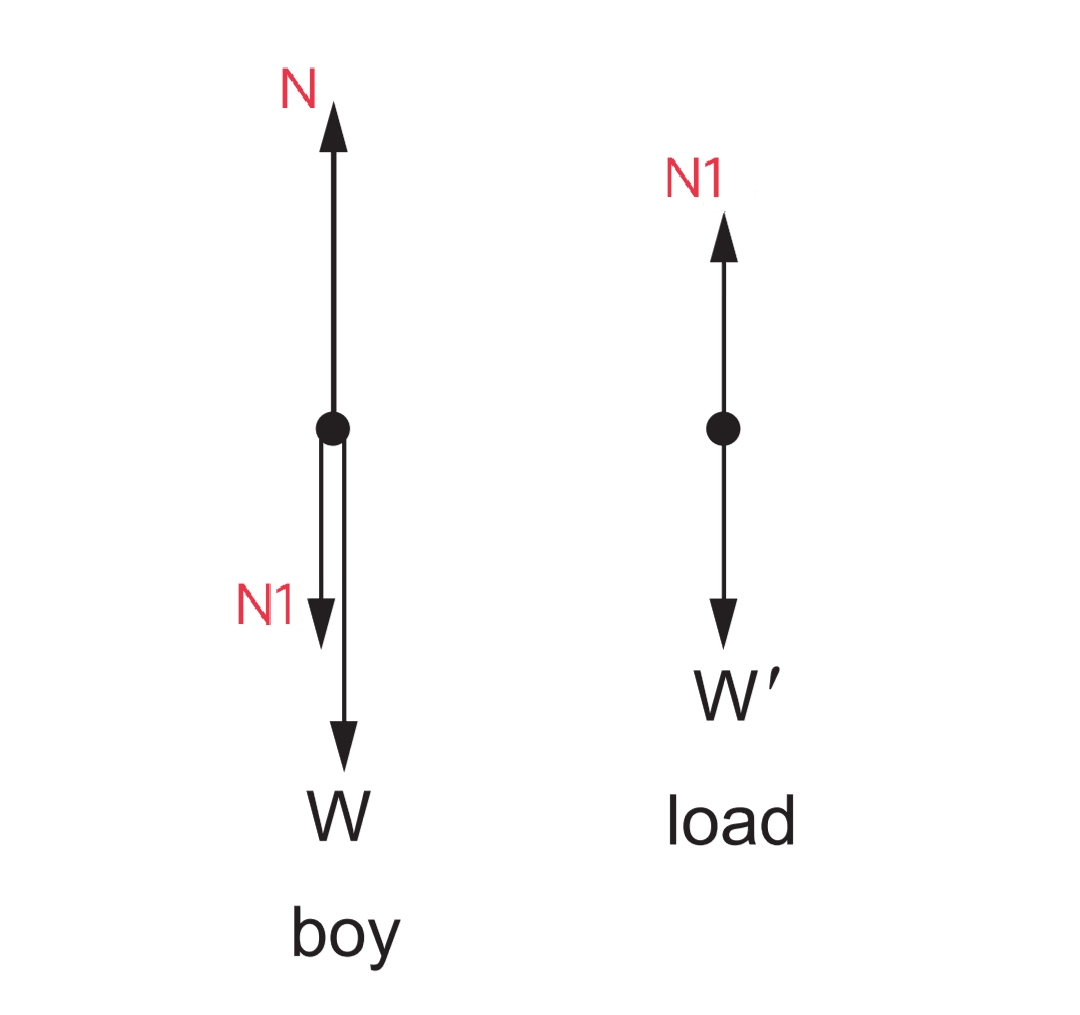# Newton's second law of motion definition

The rate of change in momentum of a body is directly proportional to the applied force on the body and takes place in the direction of the force.

The acceleration of a particle as measured from an inertial frame is given by the ( vector ) sum of all the forces acting on the particle divided by its mass.

# The formula for Newton's second law of motion

If Fis equal to force applied, a is equal to acceleration produced and m is the mass of a body then,

F = ma

Force = mass * acceleration

## Important keys for newton's second law

1. Newton's second law of motion helps to determine the magnitude of force.
1. Newton's first law is contained in Newton's second law.

# Explaining Newton's second law of motion with example

In symbols : ā = F / m or , F = ma.
The inertial frame is already defined by the first law motion. A force F acting on a particle of mass m produces an acceleration F/m in it concerning an inertial frame. This is a law of nature. If the force ceases to act at some instant, the acceleration becomes zero at the same instant.
In the equation, a and F are measured at the same instant of time.

WORKING WITH NEWTON'S FIRST AND SECOND LAW

Newton's laws refer to a particle and relate the forces acting on the particle with its acceleration and its mass. Before attempting to write an equation from Newton's law, we should very clearly understand which particle we are considering.

In any practical situation, we deal with extended bodies which are collections of a large number of particles. The laws as stated above may be used even if the object under consideration is an extended body, provided each part of this body has the same acceleration ( in magnitude and direction ). A systematic algorithm for writing equations from Newton's laws is as follows:

Step 1:
Decide the System

The first step is to decide the system on which the laws of motion are to be applied. The system may be a single particle, a block, a combination of two blocks one kept over the other, two blocks connected by a string, a piece of string, etc. The only restriction is that all parts of the system should have identical acceleration. Consider the situation shown below figure. Block B does not slip over A, the disc D slides over the string and all parts of the string are tight.A and B move together. C is not in contact with A or B. But as the length of the string between A and C does not change coma the distance moved by sea in any time interval is the same as that by A.

The same is true for G. The distance moved by G in any time interval is the same as that by A, B, or C. The direction of motion is also the same for A, B, C, and G. They have identical accelerations. We can take any of these blocks as a system or any combination of the blocks from these as a system.

Some of the examples are ( A ) , ( B ) , ( A + B ) , ( B + C ) , ( A + B + C ) , ( C + G ) , ( A + C + G ) , ( A + B + C + G ) etc. The distance covered by E is also the same as the distance covered by G but their directions are different. E moves in a vertical line whereas G in a horizontal line. ( E + G ) should not be taken as a system.

At least at this stage, we are unable to apply Newton's law treating E + G as a single particle. As the disc D slides over the string the distance covered by D is not equal to that by E in the same time interval. We should not treat D + E as a system.

Step 2 :
Identify the Forces

Once the system is decided, make a list of the forces acting on the system due to all the objects other than the system. Any force applied by the system should not be included in the list of forces. Consider the situation shown below figure.The boy stands on the floor balancing a heavy load on his head. The load presses the boy, the boy pushes the load upward the boy presses the floor downward, the floor pushes the boy upward, the earth attracts the load downward, the load attracts the earth upward, the boy attracts the earth upward and the earth attracts the boy downward. Many forces are operating in this world. Which of these forces should we include in the list of forces?

We cannot answer this question. Not because we do not know, but because we have not yet specified the system. Which is the body under consideration? Do not try to identify forces before you have decided on the system. Suppose we concentrate on the state of motion of the boy. We should then concentrate on the forces acting on the boy. The forces are listed in the upper half of the table (shown in the given image). Instead, if we take the load as the system and discuss the equilibrium of the load, the list of the forces will be different. These forces appeared in the lower half of the given table.

 System Force exertedby Magnitude of theforce Direction of theforce Nature of theforce Boy EarthfloorLoad WNN1 DownwardUpwardDownward GravitationalElectromagneticElectromagnetic Load EarthBoy W'N1 DownwardUpward GravitationalElectromagnetic

One may furnish as much information as one has about the magnitude and direction of the forces. The contact forces may have directions other than normal to the contact surface if the surfaces are rough. We shall discuss more it under the heading of friction.

Step 3:

Make a Free Body Diagram
Now, represent the system by a point in a separate diagram and draw vectors representing the forces acting on the system with this point as the common origin. The forces may lie along a line, maybe distributed in a plane ( coplanar ), or may be distributed in the space ( non - planar ).

We shall rarely encounter situations dealing with non-planar forces. For coplanar forces, the plane of the diagram represents the plane of the forces acting on the system. Indicate the magnitudes and directions of the forces in this diagram. This is called a free-body diagram. The free-body diagram for the example discussed above with the boy as the system and with the load as the system is shown image.Step 4:
Choose Axes and Write Equations

Any three mutually perpendicular directions may be chosen as the X - Y - Z axes. We give below some suggestions for choosing the axes to solve problems.

If the forces are coplanar, only two axes, say X and Y, taken in the plane of forces are needed. Choose the X-axis along the direction in which the system is known to have or is likely to have the acceleration. A direction perpendicular to it may be chosen as the Y-axis. If the system is in equilibrium, any mutually perpendicular directions in the plane of the diagram may be chosen as the axes.

Write the components of all the forces along the X-axis and equate their sum to the product of the mass of the system and its acceleration. This gives you one equation. Write the components of the forces along the Y-axis and equate the sum to zero. This gives you another equation. If the forces are collinear, this second equation is not needed.

If necessary you can go to step 1, choose another object as the system, repeat steps 2, 3, and 4 to get more equations. These are called equations of motion. Use mathematical techniques to get the unknown quantities out of these equations. This completes the algorithm The magnitudes of acceleration of different objects in a given situation are often related through kinematics. This should be properly foreseen and used together with the equations of motion.

For example, in the below image the accelerations of C and E have the same magnitudes. Equations of motion for C and E should use the same variable a for acceleration.